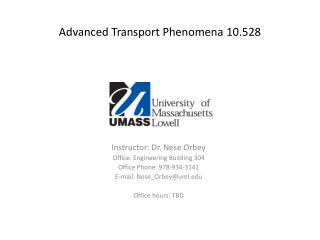DownloadDownload PresentationDownload Presentation- - - - - - - - - - - - - - - - - - - - - - - - - - - E N D - - - - - - - - - - - - - - - - - - - - - - - - - - -
Presentation Transcript

1. Advanced Transport Phenomena 10.528 Instructor: Dr. Nese Orbey Office: Engineering Building 304 Office Phone: 978-934-3141 E-mail: Nese_Orbey@uml.edu Office hours: TBD

2. Pressurized Water Reactor

3. Boiling Water Reactor

4. PEM Fuel Cell

5. Transport Phenomena • Fluid dynamics - transport of momentum • Heat transfer - transport of energy • Mass transfer - transport of mass of various chemical species

6. Why study these three phenomena together? • Occur simultaneously • Basic equations are similar solution “by analogy” • Molecular mechanisms are very closely related Viscosity, μ thermal conductivity, k Diffusivity, D

7. APPROACH • Understand the system; conceptualize 2. Apply fundamental laws Determine constitutive equations Determine boundary conditions 3. Solve the equations with boundary conditions 4. Interpret physically

8. Mathematical Formulation Fundamental Physical Principles (Basic concepts) • Conservation of mass • Conservation of chemical species • Conservation of momentum • Conservation of energy Inventory Equations Rate in –Rate out+ Rate of generation = Rate of accumulation Constitutive Equations • Newton’s Law of viscosity • Fourier’s Law • Fick’s Law

9. Levels of Transport Phenomena • Macroscopic Level • Microscopic Level • Molecular Level

10. Sir Isaac Newton 1642-1727 Jean Baptiste Joseph Fourier 1768 – 1830

11. Transport Coefficients For low Density Gases Kinetic theory of gases • Molecules are rigid and spherical • No interaction between molecules Equation 1-4-9 m:mass d:diameter K:Boltzman constant

12. Molecular InteractionsChapman -Enskog TheoryForce of attraction is related to the potential energy Lennard-Jones potential Equation 1.4-10 σ:collision diameter ε: energy of interaction Ωμ: f(KT/ε) Appendix E Table E.2